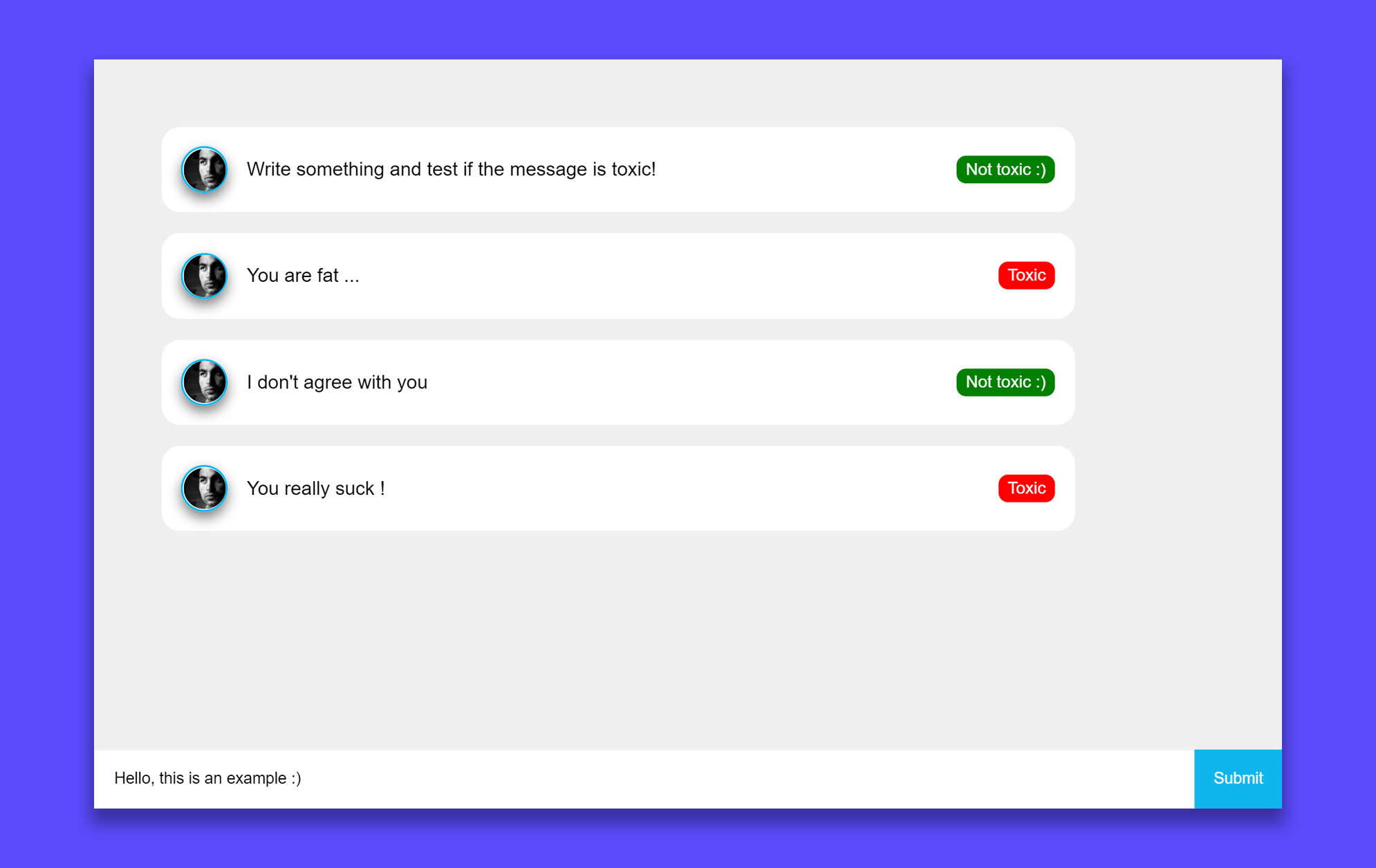# Detect toxic language with TensorFlow.js

In this tutorial I am going to show you how to detect toxic language within a React app with TensorFlow. As an example, we are going to create a simple chat. Because the goal is not to make a beautiful UI, I am going to skip the CSS part.

If you are not interested in the React part you can directly go to this section.

## Demo

See demo app or source code## Let's begin

First we need to create a new React project.

``npx create-react-app demo-toxic-chat``

Then go into the project folder.

``cd demo-toxic-chat``

And finally start the development server.

``yarn start``

To make our example work we are going to use the toxicity model of TensorFlow. The easiest way to add it into our app is by using the official CDN's.

To do so, go into the `public` folder and add the following lines in the `<head>` of the index.html file.

``````<script src="https://cdn.jsdelivr.net/npm/@tensorflow/tfjs@2.0.0/dist/tf.min.js"></script>
<script src="https://cdn.jsdelivr.net/npm/@tensorflow-models/toxicity"></script>``````

TensorFlow models can take some time to load. The model has to be loaded before the chat is displayed.

First we need to add a loader into our `App.js` file. To make this possible we are going to use a loading state with `true` as default value.

``const [loading, setLoading] = useState(true);``

When the component did mount we load the model asynchronously.

``````useEffect(() => {
const loadModel = async () => {
// 0.9 is the minimum prediction confidence.
// Display chat
};
});``````

Finally, we display a loading or chat component depending on the state. The `App.js` file will look like this:

``````import React, {useState, useEffect} from 'react';
import './App.scss';
import Chat from "./components/Chat";

let model;

function App() {

useEffect(() => {
const loadModel = async () => {
// Display chat
};
// Load model on component mount
});

return (
<div className="App">
</div>
);
}

export default App;``````

## The chat component

The next step is to create the chat component. It is composed of a message container (where the messages are displayed), a text input and a submit button.

The chat has a state containing all the messages:

``````const [messages, setMessages] = useState([
"Write something and test if the message is toxic!",
]);``````

It also has a state containing the value of the text input. When the user changes the value we save the result in the state.

``````const [input, setInput] = useState("");
const handleInputChange = (e) => setInput(e.currentTarget.value);``````

We also have to handle the addition of a new message when the form is submitted:

``````const handleSubmit = (e) => {
// Prevent submit
e.preventDefault();
// Get the current value of the input (this is our message)
const value = input;
// Clear input for the next message.
setInput("");
// Save message into the state
setMessages([...messages, value]);
};``````

The chat displays a list of messages:

``````// List of all messages
const Messages = messages.map((m, i) => (
<Message key={i} model={model} text={m} />
));``````

Finally, this is how the `Chat.js` file looks like:

``````import React, { useState } from "react";
import Message from "./Message";

const Chat = ({ model }) => {
const [messages, setMessages] = useState([
"Write something and test if the message is toxic!",
]);

const [input, setInput] = useState("");

const handleSubmit = (e) => {
// Prevent submit
e.preventDefault();
// Get input value (message)
const value = input;
// Clear input
setInput("");
// Save message into state
setMessages([...messages, value]);
};

const handleInputChange = (e) => setInput(e.currentTarget.value);

// List of all messages
const Messages = messages.map((m, i) => (
<Message key={i} model={model} text={m} />
));

return (
<div className="chat">
<div className="chat__container">{Messages}</div>
<form onSubmit={handleSubmit} className="chat__form">
<input
onChange={handleInputChange}
value={input}
className="chat__input"
type="text"
/>
<button type="submit" className="chat__submit">
Submit
</button>
</form>
</div>
);
};

export default Chat;``````

## The message component

We are going to create a component that includes the text and the toxicity of a message. In this example a message will be "toxic" or "not toxic". Note that the model from TensorFlow gives more details than just a simple true or false.

To check the toxicity we are going to create a new asynchronous function that takes the model and the message as parameters.

``````const isToxic = async (model, message) => {
// Get predictions
const predictions = await model.classify(message);
// Check if there are toxic messages in the predictions
// Match is true when the message is toxic
const toxicPredictions = predictions.filter((p) => p.results.match);
};``````

We need two states. The first one is a boolean representing the toxicity of the message. The second is the loading status, then the `isToxic()` function, being asynchronous, can take some time to return the result.

``````const [toxic, setToxic] = React.useState();

We get the toxicity of the message when the component did mount.

``````React.useEffect(() => {
const getToxic = async () => {
// Get toxicity of message
const textToxicity = await isToxic(model, text);
// Save toxicity into state
setToxic(textToxicity);
// Display toxicity
};
getToxic();
});``````

Finally, the complete `Message.js`file:

``````import React, {useState} from "react";

const isToxic = async (model, message) => {
// Get predictions
const predictions = await model.classify(message);
// Check if there are toxic messages in the predictions
// Match is true when the message is toxic
const toxicPredictions = predictions.filter((p) => p.results.match);
};

const Message = ({ text, model }) => {
const [toxic, setToxic] = useState();

React.useEffect(() => {
const getToxic = async () => {
// Get toxicity of message
const textToxicity = await isToxic(model, text);
// Save toxicity into state
setToxic(textToxicity);
// Display toxicity
};
getToxic();
});

return (
<div className="chat__message">
<span className="chat__message__text">{text}</span>
</div>
);
};

export default Message;``````

## Congratulation!

Congratulation, you created our example toxic chat. If you liked the article follow me on dev.to and check out my website.